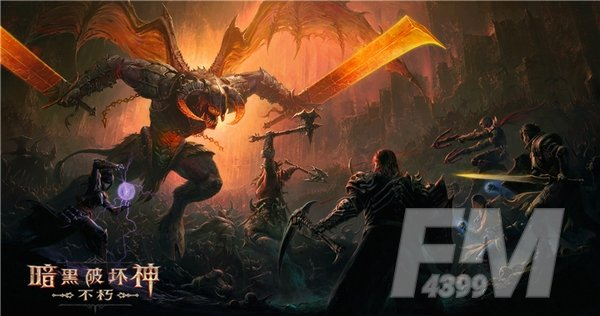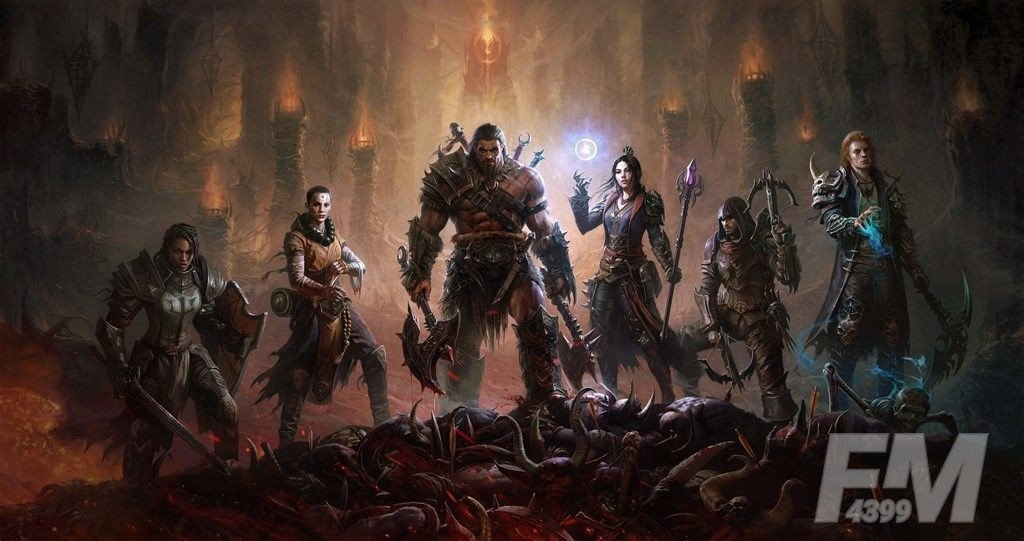# 暗黑破坏神不朽属性详情介绍 暗黑破坏神不朽属性分析讲解### 暗黑破坏神不朽属性详情介绍

1点力量=4点攻击(力量职业)=1点攻击等级=1点防御等级

1点智力=4点攻击(智力职业)=1点攻击等级=1点防御等级

1点韧性=6点护甲=6点护甲穿透=1点攻击等级=1点防御等级

1点耐力=40点生命=1点攻击等级=1点防御等级

1点精神=1点效能=1点抗性=1点攻击等级=1点防御等级